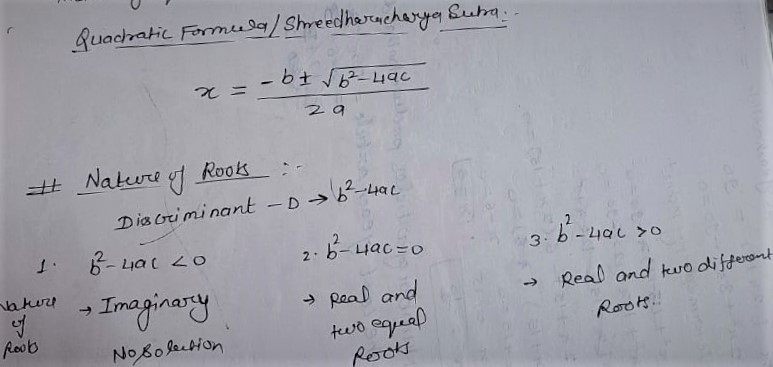# NCERT Solution Exercise 4.4 Class10

Q1.Find the roots of the Following quadratic equations, if they exist, by the method of quadratic formula.

X =[-b ± √(b² – 4ac)]/2a

Nature of Roots:

Discriminant(D)= b² – 4ac

1. b²- 4ac < 0

Nature of Roots : Imaginary/No real root

2. b² – 4ac = 0

Nature of Roots: Real and two equal Roots

3. b² – 4ac >0

Nature of Roots: Real and two different roots(i) 2x² – 3x + 5 = 0

Discriminant(D):

b² – 4ac = (-3)² – 4×2×5

⇒ b² – 4ac = 9 – 40

⇒  b² – 4ac = -31 < 0

Nature of roots: No Real and No Solution

(iii) 2x² – 6x + 3 = 0

Discriminant(D):

b²- 4ac = (-6)² – 4×2×3

⇒ b²- 4ac = 36 – 24

⇒ b²- 4ac = 12>0

Nature of roots: Two Real and Different Roots

x = [-b ± √(b² – 4ac)]/2a

⇒ x = [-(-6) ± √12]/2×2

⇒ x = [ 6 ± 2√3]/4

⇒ x = 2[ 3 ± √3]/4

x = (3+√3)/2 ; x = (3-√3)/2

x = (3+√3)/2, (3-√3)/2

(iii)

Solution:

Discriminant:

b² – 4ac = (-4√3)² – 4×3×4

⇒ b² – 4ac = 48 – 48

⇒ b² – 4ac = 0

Nature of Roots: Two Real and Equal Roots

x= [-b ± √(b² – 4ac)]/2a

⇒ x = [-(-4√3) ± √0]/2×3

⇒ x = [-(-4√3) ± √0]/6

⇒ x = 4√3 ± 0]/6

x = 4√3 – 0/6 ; x = 4√3 + 0/6

⇒ x = 4√3/6 ; x = 4√3/6

⇒ x = 2√3/3 ; x = 2√3/3

⇒ x = 2/√3 ; x = 2/√3

x = 2/√3 ,2/√3

Q.2 Find the value of k for each of the following by Quadratic Equations, so that they have two equal roots.

(i) 2x²+ k x + 3 = 0

Solution:

Condition For two real roots.

b² – 4ac = 0

⇒ k² – 4×2×3 = 0

⇒ k² – 24 = 0

⇒ k² = 24

⇒ k = ±√24

⇒ k = ±2√6

(ii) k x(x -2) + 6 = 0

Solution:

k x(x -2) + 6 = 0

⇒ k x² – 2kx + 6 = 0

Condition for two real and equal roots

b² – 4ac = 0

⇒ (2k)² – 4×k×6 = 0

⇒ 4k² – 24k = 0

⇒ 4k(k – 6) = 0

4k = 0 ; k – 6 = 0

⇒ k = 0/4 ; k = 6

⇒ k = 0 , 6

Q.3 Is it possible to design a rectangular mango  grove whose length is twice its breadth, and the area is 800 m²? If so, find its length and breadth.

Solution:

Let the Breadth of mango grove = x m  and

Length = Area of Rectangle

⇒ 2 x × x = 800

⇒ 2 x² = 800

⇒ x² = 800/2

⇒ x² = 400

⇒ x = √400

⇒ x = 20

Hence length is 2x = 2×20 = 40 m

Q.4 Is the following situation possible? If so, determine their present ages. The sum of the ages of two friends is 20 years. Four years ago, the product of their ages in years was 48.

Let the present age of 1st friend be = x years

Age of 2nd friend be = (20 – x)years

Before 4 years

1st Friend age was = (x – 4) years

2nd Friend age was = 20 – x – 4= (16 – x) years

According to condition:

Product of their Ages = 48

(x – 4)(16 – x) = 48

⇒ x(16 – x) – 4(16 – x) = 48

⇒ 16x – x² – 64 + 4x = 48

⇒ 20x – x² – 64 – 48 = 0

⇒ – x² + 20x – 112 = 0

⇒ – ( x² – 20x + 112) = 0

⇒ x² – 20x + 112 = 0

Discriminant:

D = b² – 4ac

⇒ D = (-20)² – 4×1×112

⇒ D = 400 – 448

⇒ D = -48 < 0

Nature of roots ; No real roots.

No this situation is not possible.

Q.5 Is it possible to design a rectangular park of perimeter 80 m and area 400 m²? If so, find its length and breadth.

Solution:

2( Length + Breadth ) = Perimeter of Rectangular park

⇒ 2 (L + B) = 80

⇒ L + B = 80/2

⇒ L + B = 40 ———(i)

Let Length be = x meters

⇒ x  = 40 – x

Length × Breadth = Area of Rectangular Park

⇒ x × (40 – x) = 400

⇒ 40x – x² = 400

⇒ x² – 40x + 400 = 0

⇒ x² – (20 + 20)x + 400 = 0

⇒ x² – 20x – 20x + 400 = 0

⇒ x (x – 20) – 20(x – 20) = 0

⇒ (x – 20)(x – 20) = 0

x = 20 ; x = 20

Hence Length of Park = 20 m

Breadth of Park = 20 m